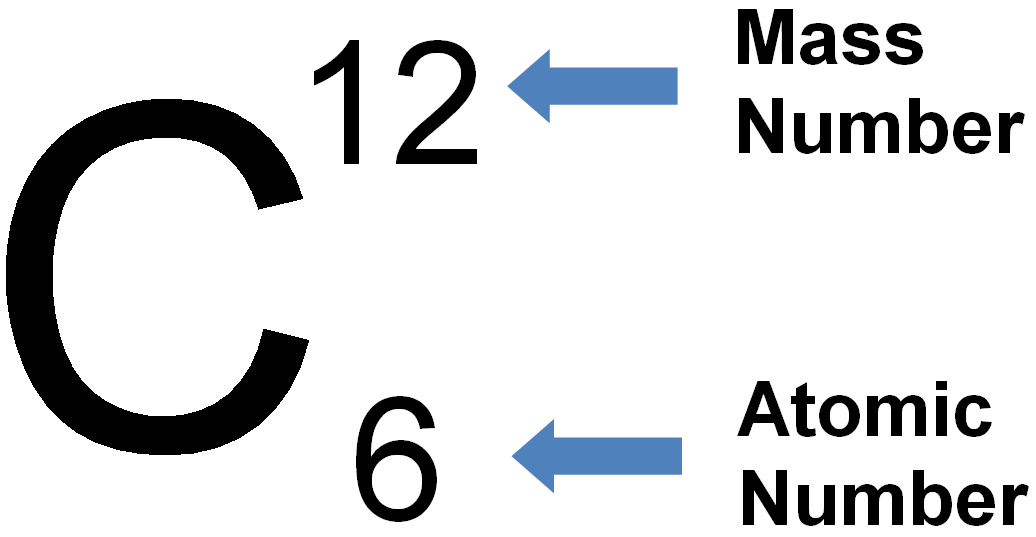# Problem: What is the mass, in grams, of 5 mol of 12C?

###### FREE Expert Solution

The mass of an element can be determined by the atomic mass of the element and the number of moles of atoms present.

The atomic mass of a substance is denoted as the mass number follows:We will solve this problem through these steps:

• Convert the number of moles of C to the mass of C using the atomic mass of 12C.
87% (95 ratings)###### Problem Details

What is the mass, in grams, of 5 mol of 12C?